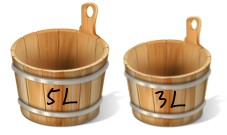## 四公升的水創造力謎題1. 把5L水桶裝滿，再從5L水桶把水倒到3L水桶裡；3L水桶到滿時，5L水桶裡剛好剩下2L的水
2. 把3L的水桶倒空，再把5L水桶裡的2L水都倒進3L水桶裡
3. 把5L水桶再次裝滿，再從5L水桶緩緩把水倒進倒到3L水桶裡，由於3L水桶裡原已有2L水，所以再倒1L就滿了，此時5L水桶裡就剛好剩下4L水

#### 解析

（以上條件單純是想避開某些太過瑣碎的情況）

A 是 24L 的水桶，B 是 31L 的水桶，

31=24*1+7 -> 7=31*1+24*(-1)
24=7*3+3 -> 3=24*1+7*(-3)
7=3*2+1 -> 1=7*1+3*(-2)

1=7*1+[24*1+7*(-3)]*(-2) -> 1=24*(-2)+7*7
1=24*(-2)+[31*1+24*(-1)]*7 -> 1=31*7+24*(-9)

25,348